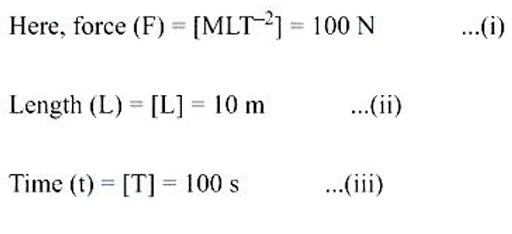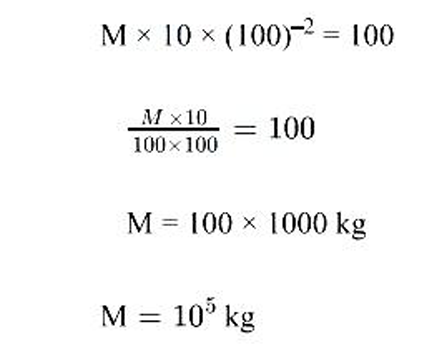# in a new system of units , the unit of force is 100 N , unit of length is 10 m and unit of time is 100s , unit of mass in this system can be expressed as

more_vert

in a new system of units , the unit of force is 100 N , unit of length is 10 m and unit of time is 100s , unit of mass in this system can be expressed as

A)105kg

B)106kg

C)102​​​​​​​kg

D)103​​​​kg

more_vert

verified

Correct option is A) 105kgSubstituting value of L and T from equation 2 and 3 in eq 1 we get